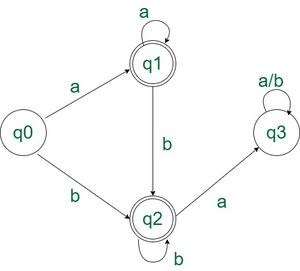Program to construct a DFA which accepts the language having all ‘a’ before all ‘b’

• Last Updated : 14 Dec, 2021

Given a string S, the task is to design a Deterministic Finite Automata (DFA) for accepting the language L = {aNbM | N ≥ 0, M ≥ 0, N+M ≥ 1}. , i.e., a regular language L such that all ‘a’ occur before the first occurrence of ‘b’ {a, ab, aab, bb…, }. If the given string follows the given language L, then print “Accepted”. Otherwise, print “Not Accepted”.

Examples

Input: S = “aabbb”
Output: Accepted
Explanation: All the ‘a’ come before ‘b’ s.

Input: S = “ba”
Output: Not Accepted
Explanation: ‘b’ comes before ‘a’.

Input: S = “aaa”
Output: Accepted
Explanation: Note that ‘b’ does not need to occur in S

Input: S = “b”
Output: Accepted
Explanation: Note that ‘a’ does not need to occur in S

Approach: The problem can be accepted only when the following cases are met:

• All the characters can be ‘a’.
• All the characters can be ‘b’.
• All the ‘b’ come occur after all the ‘a’.
• There is at least one character in the string.

This can be better visualized with the help of the state transition diagram of the DFA

State Transition Diagram:State Transition Diagram of the above DFA

Below is the implementation of the above approach:

C++

 // C++ Program to implement// the above approach#include using namespace std;  // Function for state zero Q0int startStateQ0(char s) {         int state;    if (s == 'a')        state = 1;    else if (s == 'b')        state = 2;    else        state = -1;     return state;}         // Function for first state Q1int firstStateQ1(char s) {         int state;    if (s == 'a')        state = 1;    else if (s == 'b')        state = 2;    else        state = -1;             return state;}         // Function for second state Q2int secondStateQ2(char s) {         int state;    if (s == 'b')        state = 2;    else if (s == 'a')        state = 3;    else        state = -1;             return state;}         // Function for third state Q3int thirdStateQ3(char s) {         int state = 3;    return state;}         // Function to check// if the string is accepted or notint isAcceptedString(string String) {         int l = String.length();     // dfa tells the number associated    // with the present dfa = state    int state = 0;    for (int i = 0; i < l; i++) {        if (state == 0)            state = startStateQ0(String[i]);        else if (state == 1)            state = firstStateQ1(String[i]);        else if (state == 2)            state = secondStateQ2(String[i]);        else if (state == 3)            state = thirdStateQ3(String[i]);        else            return 0;    }    if (state == 1 || state == 2)        return 1;    else        return 0;} int main() {         string String = "ba";    if (isAcceptedString(String))        cout << "ACCEPTED";    else        cout << "NOT ACCEPTED";}     // This code is contributed by Samim Hossain Mondal.

Java

 // Java Program to implement// the above approachimport java.util.*;public class GFG {     // Function for state zero Q0    static int startStateQ0(char s)    {         int state;        if (s == 'a')            state = 1;        else if (s == 'b')            state = 2;        else            state = -1;         return state;    }     // Function for first state Q1    static int firstStateQ1(char s)    {         int state;        if (s == 'a')            state = 1;        else if (s == 'b')            state = 2;        else            state = -1;         return state;    }     // Function for second state Q2    static int secondStateQ2(char s)    {         int state;        if (s == 'b')            state = 2;        else if (s == 'a')            state = 3;        else            state = -1;         return state;    }     // Function for third state Q3    static int thirdStateQ3(char s)    {         int state = 3;        return state;    }     // Function to check    // if the string is accepted or not    static int isAcceptedString(String Str)    {         int l = Str.length();         // dfa tells the number associated        // with the present dfa = state        int state = 0;        for (int i = 0; i < l; i++) {            if (state == 0)                state = startStateQ0(Str.charAt(i));            else if (state == 1)                state = firstStateQ1(Str.charAt(i));            else if (state == 2)                state = secondStateQ2(Str.charAt(i));            else if (state == 3)                state = thirdStateQ3(Str.charAt(i));            else                return 0;        }        if (state == 1 || state == 2)            return 1;        else            return 0;    }     public static void main(String args[])    {         String Str = "ba";        if (isAcceptedString(Str) != 0)            System.out.println("ACCEPTED");        else            System.out.println("NOT ACCEPTED");    }} // This code is contributed by Samim Hossain Mondal.

Python3

 # Function for state zero Q0def startStateQ0(s):    if (s == 'a'):        state = 1    elif (s == 'b'):        state = 2    else:        state = -1    return state # Function for first state Q1def firstStateQ1(s):    if (s == 'a'):        state = 1    elif (s == 'b'):        state = 2    else:        state = -1    return state # Function for second state Q2def secondStateQ2(s):    if (s == 'b'):        state = 2    elif (s == 'a'):        state = 3    else:        state = -1    return state # Function for third state Q3def thirdStateQ3(s):    state = 3    return state #Function to check#if the string is accepted or notdef isAcceptedString(String):    l = len(String)     # dfa tells the number associated    # with the present dfa = state    state = 0    for i in range(l):        if (state == 0):            state = startStateQ0(String[i])        elif (state == 1):            state = firstStateQ1(String[i])        elif (state == 2):            state = secondStateQ2(String[i])        elif (state == 3):            state = thirdStateQ3(String[i])        else:            return 0    if(state == 1 or state == 2):        return 1    else:        return 0 # Driver codeif __name__ == "__main__":     String = "ba"    if (isAcceptedString(String)):        print("ACCEPTED")    else:        print("NOT ACCEPTED")

C#

 // C# Program to implement// the above approachusing System;class GFG {     // Function for state zero Q0    static int startStateQ0(char s)    {         int state;        if (s == 'a')            state = 1;        else if (s == 'b')            state = 2;        else            state = -1;         return state;    }     // Function for first state Q1    static int firstStateQ1(char s)    {         int state;        if (s == 'a')            state = 1;        else if (s == 'b')            state = 2;        else            state = -1;         return state;    }     // Function for second state Q2    static int secondStateQ2(char s)    {         int state;        if (s == 'b')            state = 2;        else if (s == 'a')            state = 3;        else            state = -1;         return state;    }     // Function for third state Q3    static int thirdStateQ3(char s)    {         int state = 3;        return state;    }     // Function to check    // if the string is accepted or not    static int isAcceptedString(string Str)    {         int l = Str.Length;         // dfa tells the number associated        // with the present dfa = state        int state = 0;        for (int i = 0; i < l; i++) {            if (state == 0)                state = startStateQ0(Str[i]);            else if (state == 1)                state = firstStateQ1(Str[i]);            else if (state == 2)                state = secondStateQ2(Str[i]);            else if (state == 3)                state = thirdStateQ3(Str[i]);            else                return 0;        }        if (state == 1 || state == 2)            return 1;        else            return 0;    }     public static void Main()    {         string Str = "ba";        if (isAcceptedString(Str) != 0)            Console.Write("ACCEPTED");        else            Console.Write("NOT ACCEPTED");    }} // This code is contributed by ukasp.

Javascript


Output
NOT ACCEPTED

Time Complexity: O(N) where N is the length of the string
Auxiliary Space: O(1)

My Personal Notes arrow_drop_up